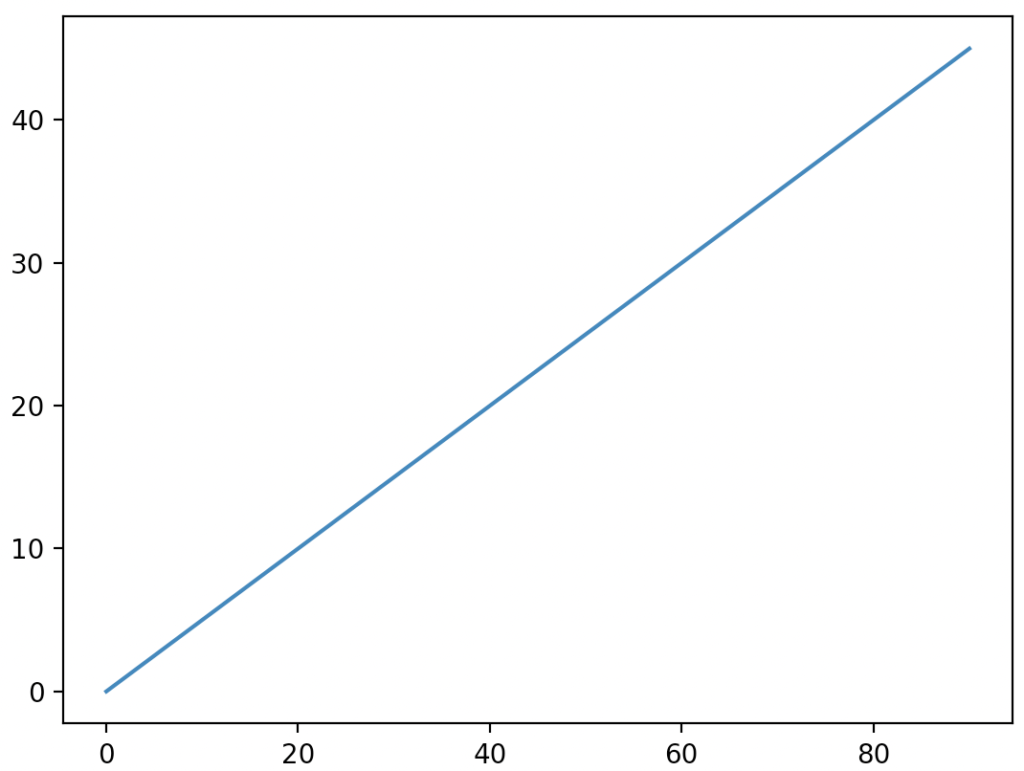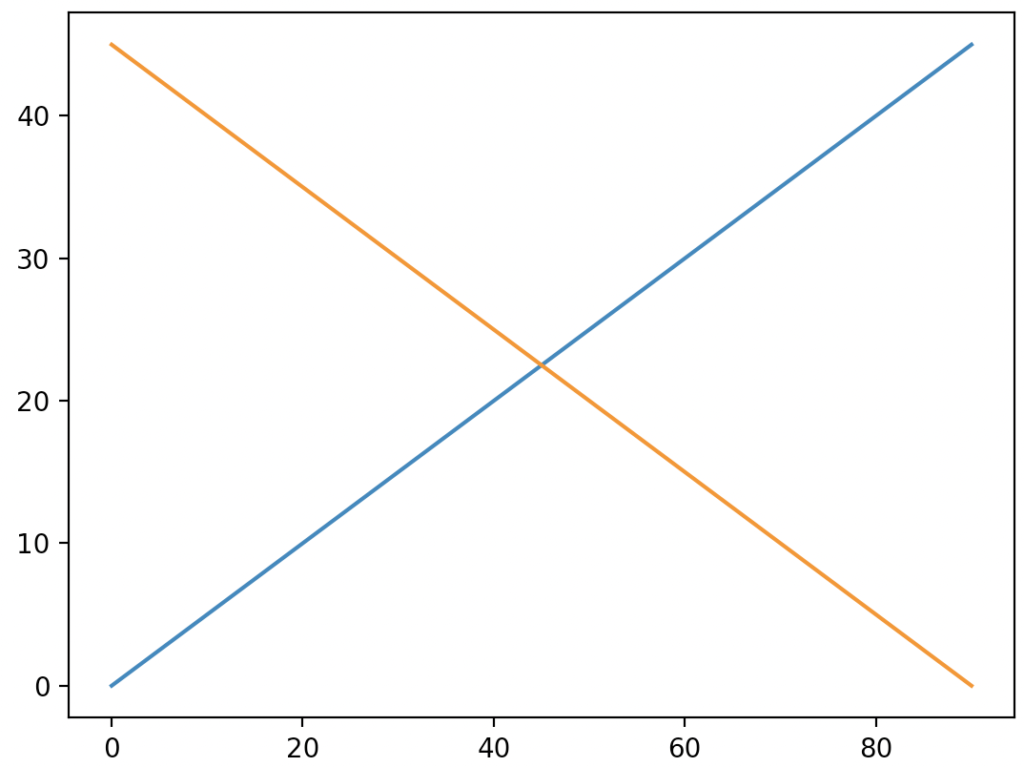# Matplotlib line

To plot a line plot in Matplotlib, you can use the “matplotlib.pyplot.plot()” function. There’s no specific lineplot() function. Instead, line plots display numerical values on one axis and categorical values on the other.

A line plot is used to display the continuous values. The points are connected using the line; hence this plot is called a line plot. The line plot can be plotted using the matplotlib.pyplot.plot() function. The plot() function creates a graph for the given points; the displayed graph is in the form of lines.

### Syntax

``matplotlib.pyplot.plot(*args, scalex=True, scaley=True, data=None, **kwargs)``

### Arguments

The matplotlib.pyplot.plot() function has one required argument and four optional arguments  as parameters:

1. args – x,y: This parameter has two values, x, and y. The points are passed in the x and y argument. This x and y can have values like numbers or a list of numbers.
2. fmt: fmt stands for format. This is an optional argument. This argument takes a string as the argument. This string represents the format in which the graph is needed to be created. For example, some color codes used in string formats are r for red, b for blue, g for green, and y for yellow. Likewise, some of the markers used in the string formats are “o” for circle, ‘.’ for point, ‘*’ for star, and “+” for plus.
3. data: It is an object with labeled data passed as an argument. This is an optional argument.

### Return value

The plot() function returns a list of 2d lines representing the plotted data. The returned list consists of points on the line graph.

### Example 1

``````# Importing matplotlib.pyplot as plt.
import matplotlib.pyplot as plt

# Importing numpy as np
import numpy as np

# create a numpy array for storing the x coordinates
x = np.arange(0, 100, 10)

# create a numpy array for storing the y coordinates
y = np.arange(0, 50, 5)

# pass the x coordinates and y coordinates into the plot() function
plt.plot(x, y)

# displaying the created graph using the show method
plt.show()``````

OutputIn this program, we imported matplotlib.pyplot for plotting the line graph. The matplotlib library consists of all the functions for plotting different types of graphs. Then we imported numpy for creating x and y coordinates.

Then, we created x and y coordinates and stored the numpy array in the x and y variables.

The x variable stores the values from 0 to 100 in intervals of 10, and the y variable stores the values from 0 to 50 in an interval of 5. Then, we passed the two coordinates into the plot() function. The plot() function plots the graph across the x and y-axis.

This generated graph is called the line graph. The output graph is a straight line. Then, the graph is displayed using the show() function.

### Example 2

To plot multiple lines in Python, you can use the “matplotlib.pyplot.plot()” function.

``````# Importing matplotlib.pyplot as plt.
import matplotlib.pyplot as plt

# Importing numpy as np
import numpy as np

# create a numpy array for storing the x coordinates
x = np.arange(0, 100, 10)

# create a numpy array for storing the y coordinates
y = np.arange(0, 50, 5)

# x and y coorindates for the second line is stored in the variable
u = np.arange(90, -10, -10)
v = np.arange(0, 50, 5)
print(u)
print(v)

# the plot function is called with coordinates x,y,u,v
plt.plot(x, y, u, v)

# displaying the created graph using the show method
plt.show()``````

OutputIn this program, we imported matplotlib.pyplot for plotting the graph. The matplotlib library consists of all the functions for plotting different types of graphs. Then, we imported numpy for creating x and y coordinates.

Then, we created x and y coordinates and stored this numpy array in x and y variables. Then, we created the x and y coordinates for the second line and stored them in the u and v variables.

Then, we called the plot() function with x and y as the first argument. Then, we passed the second line coordinates. The plot() function creates two lines in a single plot. These lines intersect with each other in their center.

This site uses Akismet to reduce spam. Learn how your comment data is processed.